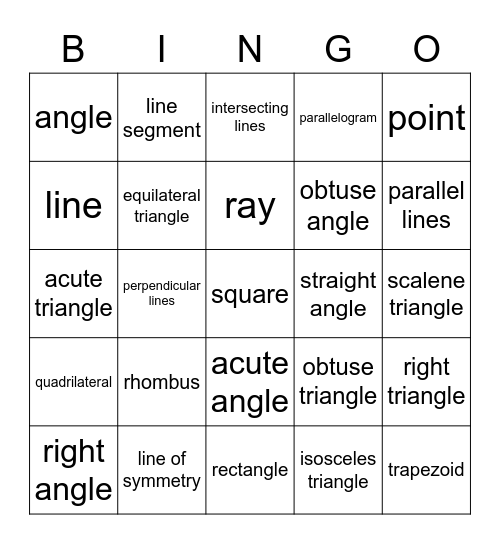# Chapter 10 BingoThis bingo card has a free space and 24 words: rhombus, line of symmetry, ray, right angle, point, angle, line, line segment, straight angle, acute angle, obtuse angle, acute triangle, obtuse triangle, right triangle, equilateral triangle, isosceles triangle, scalene triangle, intersecting lines, parallel lines, perpendicular lines, trapezoid, quadrilateral, parallelogram and rectangle.

## Play Online